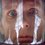# Playing with intgerals: The Length of the Function

How can we find a formula for the length of the function?

As the topic suggests we'll need integrals. First of all lets recall a well-known Pythagorean Theorem. It states that in a right triangle we have $c^2=a^2+b^2$, with $c$ denoting length of the hypotenuse and $a,b$ - lengths of catheti. Now we'll consider a very tiny bit of function, so tiny than we can aproximate the function with a line. Then according to Pythagorean Identity we'll have $s^2=x^2+y^2$, where $s$ is the length of the function and $x,y$ - $\Delta x,\Delta f(x)$ respectively. Now lets use our knowledge of derivatives to transform our identity into $ds^2=dx^2+dy^2$, which may be rearanged to get $ds=\sqrt{1+\left(\dfrac{dy}{dx}\right)^2}\,dx=\sqrt{1+[f^\prime(x)]^2}\,dx$. After integrating both sides we arrive to the desired result

$s=\displaystyle\int^b_a{\sqrt{1+[f^\prime(x)]^2}}\,dx.$Note by Nicolae Sapoval
6 years, 10 months ago

This discussion board is a place to discuss our Daily Challenges and the math and science related to those challenges. Explanations are more than just a solution — they should explain the steps and thinking strategies that you used to obtain the solution. Comments should further the discussion of math and science.

When posting on Brilliant:

• Use the emojis to react to an explanation, whether you're congratulating a job well done , or just really confused .
• Ask specific questions about the challenge or the steps in somebody's explanation. Well-posed questions can add a lot to the discussion, but posting "I don't understand!" doesn't help anyone.
• Try to contribute something new to the discussion, whether it is an extension, generalization or other idea related to the challenge.

MarkdownAppears as
*italics* or _italics_ italics
**bold** or __bold__ bold
- bulleted- list
• bulleted
• list
1. numbered2. list
1. numbered
2. list
Note: you must add a full line of space before and after lists for them to show up correctly
paragraph 1paragraph 2

paragraph 1

paragraph 2

[example link](https://brilliant.org)example link
> This is a quote
This is a quote
    # I indented these lines
# 4 spaces, and now they show
# up as a code block.

print "hello world"
# I indented these lines
# 4 spaces, and now they show
# up as a code block.

print "hello world"
MathAppears as
Remember to wrap math in $$ ... $$ or $ ... $ to ensure proper formatting.
2 \times 3 $2 \times 3$
2^{34} $2^{34}$
a_{i-1} $a_{i-1}$
\frac{2}{3} $\frac{2}{3}$
\sqrt{2} $\sqrt{2}$
\sum_{i=1}^3 $\sum_{i=1}^3$
\sin \theta $\sin \theta$
\boxed{123} $\boxed{123}$

Sort by:

My dear infinitesimal, how I hate thee!

This is best done using metric tensors, in my opinion. In fact, we define arclength using metric tensors in most contexts.

- 6 years, 10 months ago

Very nice! However, would you be able to derive it without using infinitesimals? I feel slightly uneasy, even though it is correct.

- 6 years, 10 months ago

Yep, we can do this without infinitesmals and Pythagorean Theorem. However, this approach would be a bit harder. You will need to consider the line integral, so I think this approach is a bit easier to comprehed for non-college students. If there will be more requests on altrnative solutions I will release a note discussing them (or maybe someone else will).

- 6 years, 10 months ago

How can we find a formula for the length of the function?

As the topic suggests we'll need integrals. First of all lets recall a well-known Pythagorean Theorem. It states that in a right triangle we have c2=a2+b2, with c denoting length of the hypotenuse and a,b - lengths of catheti. Now we'll consider a very tiny bit of function, so tiny than we can aproximate the function with a line. Then according to Pythagorean Identity we'll have s2=x2+y2, where s is the length of the function and x,y - Δx,Δf(x) respectively. Now lets use our knowledge of derivatives to transform our identity into ds2=dx2+dy2, which may be rearanged to get ds=1+(dydx)2−−−−−−−−−√dx=1+[f′(x)]2−−−−−−−−−√dx. After integrating both sides we arrive to the desired result

s=∫ba1+[f′(x)]2−−−−−−−−−√dx.

- 6 years, 10 months ago